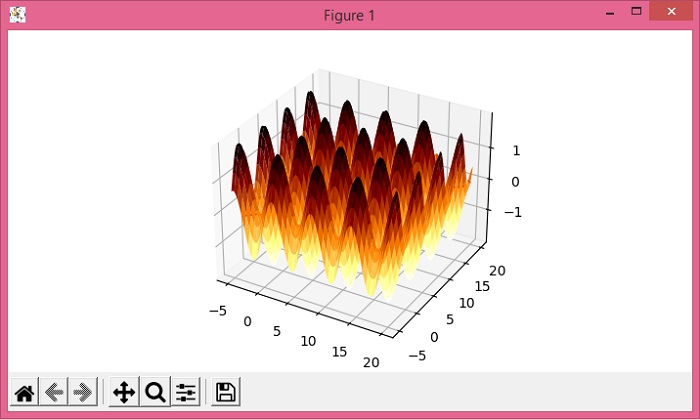# Layering a contourf plot and surface_plot in Matplotlib

MatplotlibPythonData Visualization

#### Python Data Science basics with Numpy, Pandas and Matplotlib

Most Popular

63 Lectures 6 hours

#### Data Visualization using MatPlotLib & Seaborn

11 Lectures 4 hours

#### MatPlotLib with Python

9 Lectures 2.5 hours

To layer a contourf plot and surface_plot in matplotlib, we can take the following Steps −

• Initialize the variables, delta, xrange, yrange, x and y using numpy.

• Create a new figure or activate an existing figure using figure() method.

• Get the current axis where projection='3d'.

• Create a 3d countour plot with x and y data points.

• Plot the surface with x and y data points.

• To display the figure, use show() method.

## Example

from matplotlib import pyplot as plt
import numpy as np
plt.rcParams["figure.figsize"] = [7.00, 3.50]
plt.rcParams["figure.autolayout"] = True
delta = 0.025
xrange = np.arange(-5.0, 20.0, delta)
yrange = np.arange(-5.0, 20.0, delta)
x, y = np.meshgrid(xrange, yrange)
fig = plt.figure()
ax = fig.gca(projection='3d')
ax.contour(x, y, (np.sin(x) - np.cos(y)), )
ax.plot_surface(x, y, (np.sin(x) - np.cos(y)), cmap="afmhot_r")
plt.show()

## Output